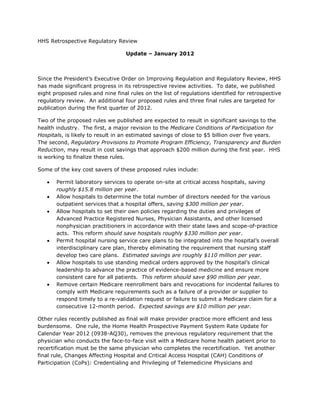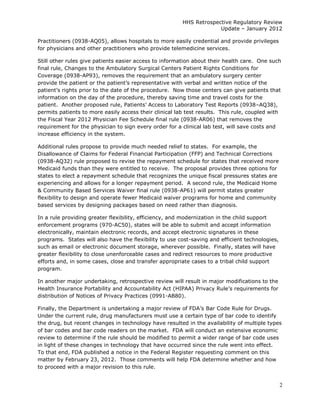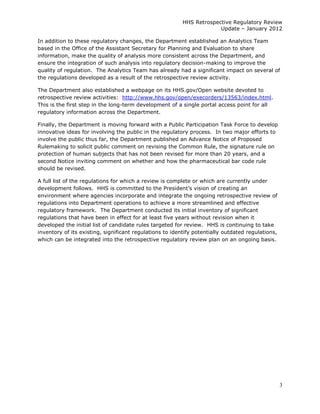Ce diaporama a bien été signalé.
Le téléchargement de votre SlideShare est en cours. ×

# Health & Human Services - Regulatory Reform - January 2012 Update

Publicité
Publicité
Publicité
Publicité
Publicité
Publicité
Publicité
Publicité
Publicité
Publicité
PublicitéPublicité
Publicité
Chargement dans…3
×

## Consultez-les par la suite

1 sur 14 Publicité

# Health & Human Services - Regulatory Reform - January 2012 Update

In January 2011, President Obama outlined his plan to create a 21st-century regulatory system – one that protects public health and welfare while promoting economic growth, innovation, competitiveness, and job creation. Among other things, his Executive Order on Regulation said the following: Always consider costs and reduce burdens for American businesses and consumers when developing rules; expand opportunities for public participation and public comment; simplify rules; promote freedom of choice; and ensure that regulations are driven by real science. this is the January 2012 update from the Department of Health and Human Services, go here for more information:

http://www.whitehouse.gov/21stcenturygov/actions/21st-century-regulatory-system

In January 2011, President Obama outlined his plan to create a 21st-century regulatory system – one that protects public health and welfare while promoting economic growth, innovation, competitiveness, and job creation. Among other things, his Executive Order on Regulation said the following: Always consider costs and reduce burdens for American businesses and consumers when developing rules; expand opportunities for public participation and public comment; simplify rules; promote freedom of choice; and ensure that regulations are driven by real science. this is the January 2012 update from the Department of Health and Human Services, go here for more information:

http://www.whitehouse.gov/21stcenturygov/actions/21st-century-regulatory-system

Publicité
Publicité

### Health & Human Services - Regulatory Reform - January 2012 Update

1. 1. HHS  Retrospective  Regulatory  Review   Update    January  2012     ,  HHS   has  made  significant  progress  in  its  retrospective  review  activities.    To  date,  we  published   eight  proposed  rules  and  nine  final  rules  on  the  list  of  regulations  identified  for  retrospective   regulatory  review.    An  additional  four  proposed  rules  and  three  final  rules  are  targeted  for   publication  during  the  first  quarter  of  2012.   Two  of  the  proposed  rules  we  published  are  expected  to  result  in  significant  savings  to  the   health  industry.    The  first,  a  major  revision  to  the  Medicare  Conditions  of  Participation  for   Hospitals,  is  likely  to  result  in  an  estimated  savings  of  close  to  \$5  billion  over  five  years.     The  second,  Regulatory  Provisions  to  Promote  Program  Efficiency,  Transparency  and  Burden   Reduction,  may  result  in  cost  savings  that  approach  \$200  million  during  the  first  year.    HHS   is  working  to  finalize  these  rules.       Some  of  the  key  cost  savers  of  these  proposed  rules  include:   Permit  laboratory  services  to  operate  on-­site  at  critical  access  hospitals,  saving   roughly  \$15.8  million  per  year.   Allow  hospitals  to  determine  the  total  number  of  directors  needed  for  the  various   outpatient  services  that  a  hospital  offers,  saving  \$300  million  per  year.   Allow  hospitals  to  set  their  own  policies  regarding  the  duties  and  privileges  of   Advanced  Practice  Registered  Nurses,  Physician  Assistants,  and  other  licensed   nonphysician  practitioners  in  accordance  with  their  state  laws  and  scope-­of-­practice   acts.    This  reform  should  save  hospitals  roughly  \$330  million  per  year.   Permit  hospital  nursing  service  care  plans  to  be  integrated  into   interdisciplinary  care  plan,  thereby  eliminating  the  requirement  that  nursing  staff   develop  two  care  plans.    Estimated  savings  are  roughly  \$110  million  per  year.   Allow  hospitals  to  use  standing  medical  orders  approved  by  the   leadership  to  advance  the  practice  of  evidence-­based  medicine  and  ensure  more   consistent  care  for  all  patients.    This  reform  should  save  \$90  million  per  year.   Remove  certain  Medicare  reenrollment  bars  and  revocations  for  incidental  failures  to   comply  with  Medicare  requirements  such  as  a  failure  of  a  provider  or  supplier  to   respond  timely  to  a  re-­validation  request  or  failure  to  submit  a  Medicare  claim  for  a   consecutive  12-­month  period.    Expected  savings  are  \$10  million  per  year.   Other  rules  recently  published  as  final  will  make  provider  practice  more  efficient  and  less   burdensome.    One  rule,  the  Home  Health  Prospective  Payment  System  Rate  Update  for   Calendar  Year  2012  (0938-­AQ30),  removes  the  previous  regulatory  requirement  that  the   physician  who  conducts  the  face-­to-­face  visit  with  a  Medicare  home  health  patient  prior  to   recertification  must  be  the  same  physician  who  completes  the  recertification.    Yet  another   final  rule,  Changes  Affecting  Hospital  and  Critical  Access  Hospital  (CAH)  Conditions  of   Participation  (CoPs):  Credentialing  and  Privileging  of  Telemedicine  Physicians  and
2. 2.     HHS  Retrospective  Regulatory  Review       Update    January  2012   Practitioners  (0938-­AQ05),  allows  hospitals  to  more  easily  credential  and  provide  privileges   for  physicians  and  other  practitioners  who  provide  telemedicine  services.       Still  other  rules  give  patients  easier  access  to  information  about  their  health  care.    One  such   final  rule,  Changes  to  the  Ambulatory  Surgical  Centers  Patient  Rights  Conditions  for   Coverage  (0938-­AP93),  removes  the  requirement  that  an  ambulatory  surgery  center   provide   prior  to  the  date  of  the  procedure.    Now  those  centers  can  give  patients  that   information  on  the  day  of  the  procedure,  thereby  saving  time  and  travel  costs  for  the   patient.    Another  proposed  rule,   AQ38),   permits  patients  to  more  easily  access  their  clinical  lab  test  results.    This  rule,  coupled  with   the  Fiscal  Year  2012  Physician  Fee  Schedule  final  rule  (0938-­AR06)  that  removes  the   requirement  for  the  physician  to  sign  every  order  for  a  clinical  lab  test,  will  save  costs  and   increase  efficiency  in  the  system.   Additional  rules  propose  to  provide  much  needed  relief  to  states.    For  example,  the   Disallowance  of  Claims  for  Federal  Financial  Participation  (FFP)  and  Technical  Corrections   (0938-­AQ32)  rule  proposed  to  revise  the  repayment  schedule  for  states  that  received  more   Medicaid  funds  than  they  were  entitled  to  receive.    The  proposal  provides  three  options  for   states  to  elect  a  repayment  schedule  that  recognizes  the  unique  fiscal  pressures  states  are   experiencing  and  allows  for  a  longer  repayment  period.    A  second  rule,  the  Medicaid  Home   &  Community  Based  Services  Waiver  final  rule  (0938-­AP61)  will  permit  states  greater   flexibility  to  design  and  operate  fewer  Medicaid  waiver  programs  for  home  and  community   based  services  by  designing  packages  based  on  need  rather  than  diagnosis.     In  a  rule  providing  greater  flexibility,  efficiency,  and  modernization  in  the  child  support   enforcement  programs  (970-­AC50),  states  will  be  able  to  submit  and  accept  information   electronically,  maintain  electronic  records,  and  accept  electronic  signatures  in  these   programs.    States  will  also  have  the  flexibility  to  use  cost-­saving  and  efficient  technologies,   such  as  email  or  electronic  document  storage,  wherever  possible.    Finally,  states  will  have   greater  flexibility  to  close  unenforceable  cases  and  redirect  resources  to  more  productive   efforts  and,  in  some  cases,  close  and  transfer  appropriate  cases  to  a  tribal  child  support   program.   In  another  major  undertaking,  retrospective  review  will  result  in  major  modifications  to  the   Health  Insurance  Portability  and  Accountability  Act  (HIPAA)  Privacy  Rule requirements  for   distribution  of  Notices  of  Privacy  Practices  (0991-­AB80).       F Bar  Code  Rule  for  Drugs.     Under  the  current  rule,  drug  manufacturers  must  use  a  certain  type  of  bar  code  to  identify   the  drug,  but  recent  changes  in  technology  have  resulted  in  the  availability  of  multiple  types   of  bar  codes  and  bar  code  readers  on  the  market.    FDA  will  conduct  an  extensive  economic   review  to  determine  if  the  rule  should  be  modified  to  permit  a  wider  range  of  bar  code  uses   in  light  of  these  changes  in  technology  that  have  occurred  since  the  rule  went  into  effect.     To  that  end,  FDA  published  a  notice  in  the  Federal  Register  requesting  comment  on  this   matter  by  February  23,  2012.    Those  comments  will  help  FDA  determine  whether  and  how   to  proceed  with  a  major  revision  to  this  rule.   2
3. 3.     HHS  Retrospective  Regulatory  Review       Update    January  2012   In  addition  to  these  regulatory  changes,  the  Department  established  an  Analytics  Team   based  in  the  Office  of  the  Assistant  Secretary  for  Planning  and  Evaluation  to  share   information,  make  the  quality  of  analysis  more  consistent  across  the  Department,  and   ensure  the  integration  of  such  analysis  into  regulatory  decision-­making  to  improve  the   quality  of  regulation.    The  Analytics  Team  has  already  had  a  significant  impact  on  several  of   the  regulations  developed  as  a  result  of  the  retrospective  review  activity.   The  Department  also  established  a  webpage  on  its  HHS.gov/Open  website  devoted  to   retrospective  review  activities:    http://www.hhs.gov/open/execorders/13563/index.html.     This  is  the  first  step  in  the  long-­term  development  of  a  single  portal  access  point  for  all   regulatory  information  across  the  Department.   Finally,  the  Department  is  moving  forward  with  a  Public  Participation  Task  Force  to  develop   innovative  ideas  for  involving  the  public  in  the  regulatory  process.    In  two  major  efforts  to   involve  the  public  thus  far,  the  Department  published  an  Advance  Notice  of  Proposed   Rulemaking  to  solicit  public  comment  on  revising  the  Common  Rule,  the  signature  rule  on   protection  of  human  subjects  that  has  not  been  revised  for  more  than  20  years,  and  a   second  Notice  inviting  comment  on  whether  and  how  the  pharmaceutical  bar  code  rule   should  be  revised.     A  full  list  of  the  regulations  for  which  a  review  is  complete  or  which  are  currently  under   environment  where  agencies  incorporate  and  integrate  the  ongoing  retrospective  review  of   regulations  into  Department  operations  to  achieve  a  more  streamlined  and  effective   regulatory  framework.    The  Department  conducted  its  initial  inventory  of  significant   regulations  that  have  been  in  effect  for  at  least  five  years  without  revision  when  it   developed  the  initial  list  of  candidate  rules  targeted  for  review.    HHS  is  continuing  to  take   inventory  of  its  existing,  significant  regulations  to  identify  potentially  outdated  regulations,   which  can  be  integrated  into  the  retrospective  regulatory  review  plan  on  an  ongoing  basis. 3
4. 4. !!"#\$%&'()*%+&,-%#\$%./01&('2#\$%-,%3 4*51&%#66#718/1'2#9:;9 !"# \$%&'() *+!,-./0 5624&0"70 /36&70;&<(36=26"' \$(2:840"30583%&20 \$'26(6=82&?0<896'%<06'0("<2<08'?,"306'7"3>826"'0@:3?&'<A0 D3"%3&<<0:=?82&<0 1"'23"40!"# +'6268269&,*:4&,+1* 1">=4&26"'0;82& 2"%&2B&30C62B08')08'26(6=82&?0(B8'%&<06'0@&'&762< 8'?08'26(6=82&?0 8((">=46<B>&'2< ! "#\$ %&'()*+(& ,-./01234"-56010-5.4-74 "A99=513D4:9010:2342::=..4E-./0123.4;"*,<429=49=FA09=641-4/9-G06=432H-921-9D4 89-/-.=649A3=4 OE0.4./=:070:49=7-9K4.2G=.4E-./0123.4R!SL(4K0330-54/=94D=29L44;OE0.49=7-9K4 ?052349A3=4A56=94 82910:0/210-54;"-8.<489-/-.=64 .=9G0:=.42.4609=:14.=9G0:=.I4JE0:E40.405:-5.0.1=514J01E41E=49=FA09=K=5147-9432H4 /AH30.E=64!%NBCN!! 0.4/2914-741E=4E-./01234:-56010-5.49A3=41E214.2G=.42//9-T0K21=3D4R&C%4 6=G=3-/K=51L4 >4?05234@A3=.4 .=9G0:=.4054E-./0123.L44@=G0.=41E=49=MA3210-541-4.121=41E21432H4.=9G0:=.4.E-A364 K0330-54/=94D=294054.2G05M.4-94RS4H0330-54-G=94S4D=29.L< H=4-77=9=64-5).01=L ?05234@A3=4OPQ ;'BCC)84>4?< B "#\$ %&'()*+(& ,-./01234"-8.489-/-.=64>4 UT:3A6=49=/-9105M47-946=21E.4-74/210=51.47-94JE-K4-53D4.-714B)/-0514J90.14 89-/-.=649A3=4 OE0.4./=:070:49=7-9K4.2G=.4E-./0123.4R&L&4K0330-54/=94D=29L44;OE0.49=7-9K4 ?052349A3=4A56=94 ?05234@A3=.4 9=.192051.4J=9=4A.=64;=LMLI4/=-/3=4/-.1)-/=9210G=3D4JE-429=4054.-714J90.14 /AH30.E=64!%NBCN!! 0.4/2914-741E=4E-./01234:-56010-5.49A3=41E214.2G=.42//9-T0K21=3D4R&C%4 6=G=3-/K=51L4 9=.192051.46=.0M5=641-4/9=G=514/210=51.479-K4/A3305M4-A14VW41AH=.42.41E=D4 K0330-54/=94D=294054.2G05M.4-94RS4H0330-54-G=94S4D=29.L< ;'BCC)84>4?< 9=:-G=9479-K425=.1E=.02<L44 ?05234@A3=4OPQ ' "#\$ %&'()*+(& ,-./01234"-8.489-/-.=64>4 @=K-G=41E=49=FA09=K=5141E214G=9H234-96=9.4KA.14H=42A1E=510:21=64J01E054C(4 89-/-.=649A3=4 OE0.4./=:070:49=7-9K4.2G=.4E-./0123.4R(%4K0330-54/=94D=29L4;OE0.49=7-9K40.4 ?052349A3=4A56=94 ?05234@A3=.4 E-A9.4JE=541E=9=40.45-4.121=432J41E2146=.0M521=.424./=:070:410K=792K=47-94 /AH30.E=64!%NBCN!! /2914-741E=4E-./01234:-56010-5.49A3=41E214.2G=.42//9-T0K21=3D4R&C%4 6=G=3-/K=51L4 1E=42A1E=510:210-54-74G=9H234-96=9.L4 K0330-54/=94D=294054.2G05M.4-94RS4H0330-54-G=94S4D=29.L< ;'BCC)84>4?< ?05234@A3=4OPQ C "#\$ %&'()*+(& ,-./01234"-8.489-/-.=64>4 @=6A:=49=MA321-9D4256470525:0234HA96=54-54E-./0123.4HD49=G0.05M41E=4 89-/-.=649A3=4 OE0.4./=:070:49=7-9K4.2G=.4E-./0123.4R'%%4K0330-54/=94D=29L4;OE0.49=7-9K4 ?052349A3=4A56=94 ?05234@A3=.4 XA1/210=514\$=9G0:=.4"-84;214YC(BLSC;H<;!<<41-4233-J4E-./0123.41-4=.12H30.E4 /AH30.E=64!%NBCN!! 0.4/2914-741E=4E-./01234:-56010-5.49A3=41E214.2G=.42//9-T0K21=3D4R&C%4 6=G=3-/K=51L4 1E=094-J54/-30:0=.49=M29605M41E=41-12345AKH=94-74609=:1-9.45==6=647-941E=4 K0330-54/=94D=294054.2G05M.4-94RS4H0330-54-G=94S4D=29.L<44OE=45==641-4 ;'BCC)84>4?< G290-A.4-A1/210=514.=9G0:=.41E21424E-./01234-77=9.L ?05234@A3=4OPQ /9-G06=4E-./0123.4J01E473=T0H0301D4-541E0.40..A=4J2.4H9-AME141-4-A94 211=510-54HD4OE=4Z-0514"-KK0..0-5425641E=4*K=90:254,-./01234 *..-:0210-5L S "#\$ %&'()*+(& ,-./01234"-8.489-/-.=64>4 *33-J4E-./0123.41-4.=141E=094-J54/-30:0=.49=M29605M41E=46A10=.42564/90G03=M=.4 89-/-.=649A3=4 OE0.4./=:070:49=7-9K4.2G=.4E-./0123.4R''%4K0330-54/=94D=29L44;OE0.49=7-9K4 ?052349A3=4A56=94 ?05234@A3=.4 -74*6G25:=64892:10:=4@=M0.1=9=64[A9.=.4;*8@[.<I48ED.0:0254*..0.1251.4 /AH30.E=64!%NBCN!! 0.4/2914-741E=4E-./01234:-56010-5.49A3=41E214.2G=.42//9-T0K21=3D4R&C%4 6=G=3-/K=51L4 ;8*.<I42564-1E=9430:=5.=645-5/ED.0:0254/92:1010-5=9.40542::-9625:=4J01E41E=094 K0330-54/=94D=294054.2G05M.4-94RS4H0330-54-G=94S4D=29.L<44OE=4/9-/-.=64 ;'BCC)84>4?< \$121=432J.42564.:-/=)-7)/92:10:=42:1.L4489-/-.=649=G0.0-5.4;214YC(BLBB<4 ?05234@A3=4OPQ 9=G0.0-5.41-41E=4#=60:234\$12774"-84J-A364H=4.==542.49=./-5.0G=41-4254 J-A364233-J47-942430:=5.=646-:1-94-74/-602190:4K=60:05=41-4.=9G=42.41E=4 0..A=4732MM=64HD4/2915=9.42564.12=E-36=9.40541E=4E-./01234:-KKA501DI4 ./=:070:233D41E=4*K=90:2548-602190:4#=60:234*..-:0210-5L ] "#\$ %&'()*+(& ,-./01234"-8.489-/-.=64>4 @=K-G=41E=49=FA09=K=5147-941E=41925./32514E-./012341E2149=:-G=9.401.4-J54 89-/-.=649A3=4 OE0.4./=:070:49=7-9K49=6A:=.4HA96=54-54E-./0123.4HD4!'%S4E-A9.4/=94D=294 ?052349A3=4A56=94 ?05234@A3=.4 -9M25.4;A.05M401.4-J541=2K<41-4E2G=41-4:-56A:1425646-:AK=5141E=4*PX4 /AH30.E=64!%NBCN!! -94RB%%I%%%4/=94D=29L44;OE0.49=7-9K40.4/2914-741E=4E-./01234:-56010-5.4 6=G=3-/K=51L4 H3--641D/=4G=9070:210-542M2054-5:=41E=41=2K42990G=.42141E=41925./32514 9A3=41E214.2G=.42//9-T0K21=3D4R&C%4K0330-54/=94D=294054.2G05M.4-94RS4 ;'BCC)84>4?< E-./0123L ?05234@A3=4OPQ H0330-54-G=94S4D=29.L< ^ "#\$ %&'()*+(& ,-./01234"-8.489-/-.=64>4 @=G0.=41E=4E-./012345A9.05M4.=9G0:=49=FA09=K=51.47-941E-.=4E-./0123.41E214A.=4 89-/-.=649A3=4 OE0.4./=:070:49=7-9K4.2G=.4E-./0123.4R!!%4K0330-54/=94D=29L44;OE0.49=7-9K4 ?052349A3=4A56=94 ?05234@A3=.4 254051=M921=64/3254-74:29=4054/9-G0605M4/210=514:29=L44_=4/9-/-.=641E2141E=4 /AH30.E=64!%NBCN!! 0.4/2914-741E=4E-./01234:-56010-5.49A3=41E214.2G=.42//9-T0K21=3D4R&C%4 6=G=3-/K=51L4 :29=4/32547-945A9.05M4.=9G0:=.4H=46=G=3-/=642564=/14:A99=5142.4/2914-741E=4 K0330-54/=94D=294054.2G05M.4-94RS4H0330-54-G=94S4D=29.L< ;'BCC)84>4?< ?05234@A3=4OPQ HA96=54-741E=45A9.05M4.12774E2G05M41-46=G=3-/41J-4:29=4/325.L ( "#\$ %&'()*+(& ,-./01234"-8.489-/-.=64>4 @=G0.=41E=49=FA09=K=51.4A56=941E=4K=60:2349=:-964.=9G0:=.4"-8.41E214 89-/-.=649A3=4 OE0.4./=:070:49=7-9K4.2G=.4E-./0123.4R&%4K0330-54/=94D=29L44;OE0.49=7-9K40.4 ?052349A3=4A56=94 ?05234@A3=.4 ./=:070:233D4/=9120541-4G=9H234-96=9.I41E=0942A1E=510:210-5I425641E=410K=792K=4 /AH30.E=64!%NBCN!! /2914-741E=4E-./01234:-56010-5.49A3=41E214.2G=.42//9-T0K21=3D4R&C%4 6=G=3-/K=51L4 HD4JE0:E41E=D4KA.14H=42A1E=510:21=6L44OE=4/9-/-.=64:E25M=.4J-A364233-J4 K0330-54/=94D=294054.2G05M.4-94RS4H0330-54-G=94S4D=29.L< ;'BCC)84>4?< ?05234@A3=4OPQ 26G25:=41E=4/92:10:=4-74=G06=5:=)H2.=64K=60:05=I42564=5.A9=4K-9=4 :-5.0.1=514:29=47-942334/210=51.L ;#(<#;; ;=>:=9:;9
5. 5. !!"#\$%&'()*%+&,-%#\$%./01&('2#\$%-,%3 4*51&%#66#718/1'2#9:;9 !"# \$%&'() *+!,-./0 5624&0"70 /36&70;&<(36=26"' \$(2:840"30583%&20 \$'26(6=82&?0<896'%<06'0("<2<08'?,"306'7"3>826"'0@:3?&'<A0 D3"%3&<<0:=?82&<0 1"'23"40!"# +'6268269&,*:4&,+1* 1">=4&26"'0;82& 2"%&2B&30C62B08')08'26(6=82&?0(B8'%&<06'0@&'&762< 8'?08'26(6=82&?0 8((">=46<B>&'2< & "#\$ %&'()*+(& ,-./01234"-8.489-/-.=64>4 `/621=41E=4057=:10-54:-519-34"-841-42664245=J4.125629647-940573A=5a24 89-/-.=649A3=4 OE0.4./=:070:49=7-9K4.2G=.4E-./0123.4R]L]4K0330-54/=94D=29L44;OE0.49=7-9K4 ?052349A3=4A56=94 ?05234@A3=.4 G2::05210-5L44`/621=49=MA3210-5.41-49=73=:14:A99=514.121=4-741E=42914/92:10:=.4 /AH30.E=64!%NBCN!! 0.4/2914-741E=4E-./01234:-56010-5.49A3=41E214.2G=.42//9-T0K21=3D4R&C%4 6=G=3-/K=51L4 25641=9K05-3-MDL K0330-54/=94D=294054.2G05M.4-94RS4H0330-54-G=94S4D=29.L< ;'BCC)84>4?< ?05234@A3=4OPQ !% "#\$ %&'()*+&] @=MA321-9D489-G0.0-5.41-4 @=K-G=49=MA3210-5.40..A=64/90-941-41E=4#=60:29=I4#=60:206I42564\$",V84 89-/-.=649A3=4 OE0.4./=:070:49=7-9K49=6A:=.4:-57A.0-5L44;OE0.49=7-9K40.4/2914-741E=49A3=4 ?052349A3=4A56=94 89-K-1=489-M92K4U770:0=5:DI4 P=5=701.4VK/9-G=K=514256489-1=:10-54*:14-74B%%%4;PV8*<I42//30:2H3=41-4 /AH30.E=64!%NBCN!! 1-4/9-K-1=4/9-M92K4=770:0=5:DI41925./29=5:DI42564HA96=549=6A:10-541E214 6=G=3-/K=51L4 O925./29=5:D42564PA96=54 050102346=1=9K05210-5.425642//=23.47-94#=60:29=48291.4*42564PI49=./=:10G=3DL444 J0334.2G=42.4KA:E42.4RB%%4K0330-540541E=4709.14D=29L< @=6A:10-54 ?05234@A3=4OPQ ;&%^%)84>4?< !! "#\$ %&'()*+&] @=MA321-9D489-G0.0-5.41-4 @=K-G=41E=4-A1621=64:A99=514325MA2M=40541E=4#=60:20649=MA3210-5.42564 89-/-.=649A3=4 OE0.4./=:070:49=7-9K4A/621=.4-H.-3=1=4057-9K210-5L44OE0.4J2.423.-424 ?052349A3=4A56=94 89-K-1=489-M92K4U770:0=5:DI4 9=/32:=4014J01E41E=4A/621=64#=60:29=4/=9.-55=34FA23070:210-5.47-94/ED.0:234 /AH30.E=64!%NBCN!! 9=:-KK=56210-549=:=0G=6479-K4OE=4*K=90:254X::A/210-5234OE=92/D4 6=G=3-/K=51L4 O925./29=5:D42564PA96=54 1E=92/0.1.42564-::A/210-52341E=92/0.1.4A56=94CB4"?@4YC(CLCL4 *..-:0210-5.I4V5:L4;*XO*<L44;OE0.49=7-9K40.4/2914-741E=49A3=41-4/9-K-1=4 @=6A:10-54 ?05234@A3=4OPQ /9-M92K4=770:0=5:DI41925./29=5:DI4>4HA96=549=6A:10-541E214J0334.2G=42.4 KA:E42.4RB%%4K0330-540541E=4709.14D=29L< ;&%^%)84>4?< !B "#\$ %&'()*+&] @=MA321-9D489-G0.0-5.41-4 "A99=5149=MA3210-5.430K0141E=4V51=9K=6021=4"29=4?2:03010=.47-941E=4#=51233D4 89-/-.=649A3=4 OE0.4./=:070:49=7-9K49=6A:=.4:-57A.0-5425641E=4=T1=514-74/2/=9J-947-94 ?052349A3=4A56=94 89-K-1=489-M92K4U770:0=5:DI4 @=1296=64;V"?N#@<4/9-G06=942M9==K=51.4A56=94#=60:20641-4255A23410K=4 /AH30.E=64!%NBCN!! .121=.49=321=641-41E=4:A99=514/9-:=..L44;OE0.49=7-9K40.4/2914-741E=49A3=41-4 6=G=3-/K=51L4 O925./29=5:D42564PA96=54 30K01.L444_=4/9-/-.=64=30K052105M41E=410K=430K01=642M9==K=5147-94V"?.42564 /9-K-1=4/9-M92K4=770:0=5:DI41925./29=5:DI42564HA96=549=6A:10-541E214 @=6A:10-54 9=/32:05M4014J01E41E=4.0K032949=FA09=K=5147-94.033=645A9.05M472:03010=.42564 ?05234@A3=4OPQ J0334.2G=42.4KA:E42.4RB%%4K0330-540541E=4709.14D=29L< 5A9.05M472:03010=.4;0L=LI4.121=J06=42G=92M=4-74!B4K-51E.4J01E45-4K-9=41E25424 ;&%^%)84>4?< !S)K-51E4051=9G234H=1J==54.A9G=D.<L !' "#\$ %&'()*+&] @=MA321-9D489-G0.0-5.41-4 U30K0521=41E=4=59-33K=514H294JE0:E4/9=:3A6=.49=)=59-33K=514-74/9-G06=9.42564 89-/-.=649A3=4 OE0.4./=:070:49=7-9K405:9=2.=.473=T0H0301D47-94/9-G06=9.42564.A//30=9.L44OE=4 ?052349A3=4A56=94 89-K-1=489-M92K4U770:0=5:DI4 .A//30=9.4JE-4E2G=45-149=./-56=6410K=3D41-49=)G2306210-54-94-1E=949=FA=.1.4 /AH30.E=64!%NBCN!! -G=92334/2/=9J-94HA96=54J-A3645-14:E25M=L4OE0.4./=:070:49=7-9K4 6=G=3-/K=51L4 O925./29=5:D42564PA96=54 7-94057-9K210-5L44@=G0.=41E=49=MA3210-541E214/9-G06=.47-949=G-:210-54-74 /9-6A:=.424.2G05M.4-742//9-T0K21=3D4R!%4K0330-54/=94D=29L44;OE0.49=7-9K4 @=6A:10-54 #=60:29=4H03305M4/90G03=M=.41-4=T/9=..3D4/9-G06=41E2141E=49=)=59-33K=514H294 ?05234@A3=4OPQ 0.4/2914-741E=49A3=41-4/9-K-1=4/9-M92K4=770:0=5:DI41925./29=5:DI42564 J03345-142//3D40741E=49=G-:210-540.4H2.=64A/-541E=47203A9=4-7424/9-G06=94-94 HA96=549=6A:10-541E214J0334.2G=42.4KA:E42.4RB%%4K0330-540541E=4709.14 ;&%^%)84>4?< .A//30=941-49=./-56410K=3D41-4249=)G2306210-549=FA=.1L44 D=29L< !C "#\$ %&'()*+&] @=MA321-9D489-G0.0-5.41-4 @=K-G=41E=49=MA3210-541E2149=FA09=.4#=60:29=4H03305M4/90G03=M=.41-4H=4 89-/-.=649A3=4 OE0.4./=:070:49=7-9K4.2G=.4:-33=:10-54-74057-9K210-54:-.1.47-94#=60:29=) ?052349A3=4A56=94 89-K-1=489-M92K4U770:0=5:DI4 9=G-=64074#=60:29=4:320K.429=45-14.AHK011=647-9424:-5.=:A10G=4!B)K-51E4 /AH30.E=64!%NBCN!! =59-33=64/ED.0:025.425645-5)/ED.0:0254/92:1010-5=9.41-12305M4 6=G=3-/K=51L4 O925./29=5:D42564PA96=54 /=90-6L4 2//9-T0K21=3D4RB]L^4K0330-54/=94D=29L44;OE0.49=7-9K40.4/2914-741E=49A3=41-4 @=6A:10-54 ?05234@A3=4OPQ /9-K-1=4/9-M92K4=770:0=5:DI41925./29=5:DI42564HA96=549=6A:10-541E214 J0334.2G=42.4KA:E42.4RB%%4K0330-540541E=4709.14D=29L< ;&%^%)84>4?< !S "#\$ %&'()*+&] @=MA321-9D489-G0.0-5.41-4 #-607D4CB4"?@4C&CL]%;=<4J01E4254266010-5234/9-G0.0-541E214=T=K/1.4:=912054 89-/-.=649A3=4 OE0.4./=:070:49=7-9K49=.A31.405424-5=410K=4:-.14.2G05M.4-742//9-T0K21=3D4 ?052349A3=4A56=94 89-K-1=489-M92K4U770:0=5:DI4 U564\$12M=4@=5234Q0.=2.=4;U\$@Q<472:03010=.479-K4./=:070:49=FA09=K=51.40541E=4 /AH30.E=64!%NBCN!! R!%(L^4K0330-541-4U\$@Q472:03010=.L44;OE0.49=7-9K40.4/2914-741E=49A3=41-4 6=G=3-/K=51L4 O925./29=5:D42564PA96=54 B%%%4=6010-54-741E=4b07=4\$27=1D4"-6=4;b\$"<4-741E=4[210-5234?09=489-1=:10-54 /9-K-1=4/9-M92K4=770:0=5:DI41925./29=5:DI42564HA96=549=6A:10-541E214 @=6A:10-54 *..-:0210-54;[?8*<I42.43-5M42.41E=D46=K-5.1921=4.AH.12510234:-K/3025:=4 ?05234@A3=4OPQ J0334.2G=42.4KA:E42.4RB%%4K0330-540541E=4709.14D=29L< J01E41E=4-1E=942//30:2H3=4b\$"49=FA09=K=51.4-74[?8*4!%!I4B%%%4=6010-5L ;&%^%)84>4?< 9#(<#;; ;=>:=9:;9
6. 6. !!"#\$%&'()*%+&,-%#\$%./01&('2#\$%-,%3 4*51&%#66#718/1'2#9:;9 !"# \$%&'() *+!,-./0 5624&0"70 /36&70;&<(36=26"' \$(2:840"30583%&20 \$'26(6=82&?0<896'%<06'0("<2<08'?,"306'7"3>826"'0@:3?&'<A0 D3"%3&<<0:=?82&<0 1"'23"40!"# +'6268269&,*:4&,+1* 1">=4&26"'0;82& 2"%&2B&30C62B08')08'26(6=82&?0(B8'%&<06'0@&'&762< 8'?08'26(6=82&?0 8((">=46<B>&'2< !] "#\$ %&'()*+&] @=MA321-9D489-G0.0-5.41-4 @=K-G=41E=4-H.-3=1=49=FA09=K=5141E214*KHA321-9D4\$A9M0:234"=51=9.4;*\$".<4 89-/-.=649A3=4 OE0.4./=:070:49=7-9K49=6A:=.49=6412/=47-94*\$".L44;OE0.49=7-9K40.4/2914-74 ?052349A3=4A56=94 89-K-1=489-M92K4U770:0=5:DI4 =.12H30.E4254057=:10-54:-519-34/9-M92KL /AH30.E=64!%NBCN!! 1E=49A3=41-4/9-K-1=4/9-M92K4=770:0=5:DI41925./29=5:DI42564HA96=54 6=G=3-/K=51L4 O925./29=5:D42564PA96=54 9=6A:10-541E214J0334.2G=42.4KA:E42.4RB%%4K0330-540541E=4709.14D=29L< @=6A:10-54 ?05234@A3=4OPQ ;&%^%)84>4?< !^ "#\$ %&'()*+&] @=MA321-9D489-G0.0-5.41-4 @=K-G=41E=46A/30:21=4/9-G0.0-541E214.121=.41E2141E=4M-G=9505M4H-6D4-74254 89-/-.=649A3=4 OE0.4./=:070:49=7-9K49=6A:=.49=6412/=47-94X8X.L44;OE0.49=7-9K40.4/2914-74 ?052349A3=4A56=94 89-K-1=489-M92K4U770:0=5:DI4 X9M25489-:A9=K=514X9M250a210-5.4;X8X<4E2.47A3343=M2349=./-5.0H0301D42564 /AH30.E=64!%NBCN!! 1E=49A3=41-4/9-K-1=4/9-M92K4=770:0=5:DI41925./29=5:DI42564HA96=54 6=G=3-/K=51L4 O925./29=5:D42564PA96=54 2A1E-901D47-941E=4K252M=K=5142564/9-G0.0-54-742334X8X4.=9G0:=.42564KA.14 9=6A:10-541E214J0334.2G=42.4KA:E42.4RB%%4K0330-540541E=4709.14D=29L<44 @=6A:10-54 6=G=3-/42564-G=9.==41E=40K/3=K=51210-54-741E=4/-30:0=.42564/9-:=6A9=.4 ?05234@A3=4OPQ 5=:=..29D41-4=5.A9=41E=4=77=:10G=426K050.19210-54-741E=4X8XL ;&%^%)84>4?< !( "#\$ %&'()*+&] @=MA321-9D489-G0.0-5.41-4 @=G0.=4:=9120546=705010-5.47-94X8X.41-4H=4:-5.0.1=514J01E4:A99=5146=705010-5.4 89-/-.=649A3=4 OE0.4./=:070:49=7-9K4A/621=.4-H.-3=1=4057-9K210-5L44;OE0.49=7-9K40.4/2914 ?052349A3=4A56=94 89-K-1=489-M92K4U770:0=5:DI4 A.=640541E=4X8X425641925./32514:-KKA5010=.L44 /AH30.E=64!%NBCN!! -741E=49A3=41-4/9-K-1=4/9-M92K4=770:0=5:DI41925./29=5:DI42564HA96=54 6=G=3-/K=51L4 O925./29=5:D42564PA96=54 9=6A:10-541E214J0334.2G=42.4KA:E42.4RB%%4K0330-540541E=4709.14D=29L< @=6A:10-54 ?05234@A3=4OPQ ;&%^%)84>4?< !& "#\$ %&'()*+&] @=MA321-9D489-G0.0-5.41-4 89-/-.=649A3=4 OE0.4./=:070:49=7-9K49=6A:=.49=6412/=47-94*\$".425649=.A31.405424-5=410K=4 ?052349A3=4A56=94 89-K-1=489-M92K4U770:0=5:DI4 /AH30.E=64!%NBCN!! .2G05M.4-74R!(LS4K0330-541-4*\$".L44;OE0.49=7-9K40.4/2914-741E=49A3=41-4 6=G=3-/K=51L4 O925./29=5:D42564PA96=54 M-G=9505M4H-6D41-46=G=3-/4J9011=54/-30:0=.42564/9-:=6A9=.I47-33-J05M4 /9-K-1=4/9-M92K4=770:0=5:DI41925./29=5:DI42564HA96=549=6A:10-541E214 @=6A:10-54 2::=/1=64.1256296.4-74/92:10:=I4./=:07D05M41E=41D/=I4FA25101DI4=1:LI4-74 ?05234@A3=4OPQ J0334.2G=42.4KA:E42.4RB%%4K0330-540541E=4709.14D=29L< =K=9M=5:D4=FA0/K=51I4K=60:210-5.I42564.A//30=.41E214KA.14H=40KK=6021=3D4 ;&%^%)84>4?< 2G2032H3=41-4E2563=4051=9)4-94/-.1)-/=9210G=4=K=9M=5:0=.L B% "#\$ %&'()*+&] @=MA321-9D489-G0.0-5.41-4 [=J4,=231E4V5.A925:=48-912H0301D42564*::-A512H0301D4*:14-74!&&]4;,V8**<4 89-/-.=649A3=4 OE0.4./=:070:49=7-9K4A/621=.4-A1621=649=MA3210-5.L44;OE0.49=7-9K40.4/2914 ?052349A3=4A56=94 89-K-1=489-M92K4U770:0=5:DI4 K-6070:210-5.41-41E=4,V8**41925.2:10-5.4[210-5234"-A5:0347-9489=.:90/10-54 /AH30.E=64!%NBCN!! -741E=49A3=41-4/9-K-1=4/9-M92K4=770:0=5:DI41925./29=5:DI42564HA96=54 6=G=3-/K=51L4 O925./29=5:D42564PA96=54 Q9AM489-M92K.4;["8Q8<4O=3=:-KKA50:210-5.4\$12562964G=9.0-54QL%425641E=4 9=6A:10-541E214J0334.2G=42.4KA:E42.4RB%%4K0330-540541E=4709.14D=29L<44 @=6A:10-54 *\$"4c!B4\$1256296.47-94U3=:19-50:4Q2124V51=9:E25M=4O=:E50:234@=/-914OD/=4'4 ?05234@A3=4OPQ P2.=64-542G2032H3=4057-9K210-5I4J=4H=30=G=41E=9=4J0334H=4.A//-9147-941E0.4 ,=231E4"29=4U30M0H0301D4P=5=7014V5FA09D42564@=./-5.=4;B^%NB^!<<4J=514051-4 :E25M=479-K4056A.19D4H=:2A.=41E=D4E2G=4239=26D4.E-J54.A//-9147-94 ;&%^%)84>4?< =77=:14-54Z25A29D4!I4B%!BL44"A99=514=)/9=.:90H05M4.1256296.4A.=41E=4 26-/105M41E=4:E25M=.41-41E=.=41J-4,V8**41925.2:10-5.L -A1621=64G=9.0-5.4-741E=.=41925.2:10-5.L44_=4J-A3649=109=41E=4-36=94G=9.0-5.4 -74=)/9=.:90H05M41925.2:10-5.4256426-/141E=45=J=94G=9.0-5.41-4H=4054 :-K/3025:=4J01E41E=4:A99=514=)/9=.:90H05M4.1256296.47-94#=60:29=482914QL4 B! "#\$ %&'()*+&] @=MA321-9D489-G0.0-5.41-4 @=K-G=479-K41E=4"-6=4-74?=6=9234@=MA3210-5.4;"?@<41E=4X770:=4-74 89-/-.=649A3=4 OE0.4./=:070:49=7-9K49=K-G=.4A55=:=..29D4057-9K210-5479-K41E=4"?@L44 ?052349A3=4A56=94 89-K-1=489-M92K4U770:0=5:DI4 #252M=K=5142564PA6M=14;X#P<4:-519-345AKH=9.I42//9-G2345AKH=9.I42564 /AH30.E=64!%NBCN!! ;OE0.49=7-9K40.4/2914-741E=49A3=41-4/9-K-1=4/9-M92K4=770:0=5:DI4 6=G=3-/K=51L4 O925./29=5:D42564PA96=54 057-9K210-54:-33=:10-5.4.05:=41E=D429=4-H.-3=1=42564:254H=42::=..=64-541E=4 1925./29=5:DI42564HA96=549=6A:10-541E214J0334.2G=42.4KA:E42.4RB%%4 @=6A:10-54 X#P4J=H.01=L ?05234@A3=4OPQ K0330-540541E=4709.14D=29L<44OE=9=430=3D4J-A364H=45-4-//-.010-541-4 9=K-G05M41E=4:E291479-K41E=4"?@42.4J=4E2G=4E2645-405FA090=.42H-A141E=4 ;&%^%)84>4?< :E2914.05:=4014J2.4266=64K25D4D=29.42M-L44 BB "#\$ %&'()*+(] "-5192:14d=294B%!'482914"4>4 @=K-G=49=FA09=K=5141E21424E=231E4K2051=525:=4-9M250a210-54;,#X<4-94 89-/-.=649A3=4 OE0.4./=:070:49=7-9K49=6A:=.49=6412/=47-94,#X.42564"#8.L ?052349A3=4A56=94 Q489-/-.=64>4?05234@A3=.4 :-K/=1010G=4K=60:234/3254;"#8<41E2146-=.45-14051=5641-49=5=J401.4:-5192:14 /AH30.E=64 6=G=3-/K=51L KA.145-107D41E=4M=5=9234/AH30:42143=2.14'%462D.4H=7-9=41E=4=564-741E=4 !%N!!NB%!! ;C!S^)84>4?< :-5192:14/=90-6I4HD4/AH30.E05M4245-10:=4054-5=4-94K-9=45=J./2/=9.4-74 O29M=147-947052349A3=4 /AH30:210-5e44'NB%!B >#(<#;; ;=>:=9:;9
7. 7. !!"#\$%&'()*%+&,-%#\$%./01&('2#\$%-,%3 4*51&%#66#718/1'2#9:;9 !"# \$%&'() *+!,-./0 5624&0"70 /36&70;&<(36=26"' \$(2:840"30583%&20 \$'26(6=82&?0<896'%<06'0("<2<08'?,"306'7"3>826"'0@:3?&'<A0 D3"%3&<<0:=?82&<0 1"'23"40!"# +'6268269&,*:4&,+1* 1">=4&26"'0;82& 2"%&2B&30C62B08')08'26(6=82&?0(B8'%&<06'0@&'&762< 8'?08'26(6=82&?0 8((">=46<B>&'2< B' "#\$ %&'()*8]! #=60:2064,-K=4>4"-KKA501D4 U30K0521=4243-5M.125605M4?=6=9234H2990=941-4:9=2105M4-5=4#=60:2064,-K=42564 89-/-.=649A3=4 OE=9=429=45-4.0M5070:2514=:-5-K0:4=77=:1.42..-:021=64J01E41E0.49=7-9Kf4014 ?052349A3=4A56=94 P2.=64\$=9G0:=.4_20G=94?05234 "-KKA501D)H2.=64J20G=941E214.=9G=.4KA310/3=4129M=14M9-A/.405.1=264-741E9==4 /AH30.E=64CN!SNB%!! .19=2K305=.4254=T0.105M4J20G=94/9-:=..42564/9-G06=.47-94K2T0KAK4 6=G=3-/K=51L @A3= .=/2921=N60.105:14J20G=9.L44U52H3=4.121=.41-46=.0M542564-/=921=47=J=94J20G=94 73=T0H0301DL44 /9-M92K.4HD46=.0M505M4/2:2M=.4H2.=64-545==64921E=941E254602M5-.0.L44OE0.4 O29M=147-947052349A3=4 ;BB&])?< J0334233-J4K2T0KAK473=T0H0301D47-94.121=.41-4.=9G=4KA310/3=4129M=14/-/A3210-5.4 /AH30:210-5e44OPQ 05424.05M3=4,-K=42564"-KKA501D4P2.=64\$=9G0:=.4;,"P\$<4J20G=9I49=M2963=..4 -74602M5-.0.L BC "#\$ %&'()*+'B Q0.233-J25:=4-74"320K.47-94 @=G0.=41E=49=/2DK=514.:E=6A3=4/9-G0605M41E9==4-/10-5.47-94.121=.4=3=:105M424 89-/-.=649A3=4 OE0.4./=:070:49=7-9K405:9=2.=.473=T0H0301D47-94.121=.42564/9-G06=.47-9424 ?052349A3=4A56=94 ?=6=9234?0525:023482910:0/210-54 9=/2DK=514.:E=6A3=405:3A605M4.:E=6A3=.41E2149=:-M50a=41E=4A50FA=470.:234 /AH30.E=64(N'NB%!! K-9=4=T1=56=649=/2DK=514.:E=6A3=4HD4233-J05M4.121=.41-4.=3=:142K-5M4 6=G=3-/K=51L ;??8<42564O=:E50:234 /9=..A9=.4-74.121=.41E21429=4=T/=90=5:05M4=:-5-K0:460.19=..L4 1E9==4-/10-5.47-949=/2D05M47=6=9234-G=9/2DK=51.L44"2.E4.192//=64.121=.4 "-99=:10-5.489-/-.=64>4?05234 O29M=147-947052349A3=4 J0334H=5=701479-K4243-5M=941=9K49=/2DK=514-/10-5L @A3=.4 /AH30:210-5e44^NB%!B ;BB&B)84>4?< BS "#\$ %&'()*+'] ,-K=4,=231E4?2:=)1-)?2:=4 @=G0.=41E=4#=60:2064E-K=4E=231E4.=9G0:=46=705010-542.49=FA09=64HD4.=:10-54 89-/-.=649A3=4 OE=9=4J0334H=4254=.10K21=64R'S%4K0330-54.2G05M.479-K4B%!%41-4B%!C41-4 ?052349A3=4A56=94 89-/-.=64>4?05234@A3=.4 ]C%^4-741E=4*77-962H3=4"29=4*:141-42664249=FA09=K=5141E214/ED.0:025.4 /AH30.E=64^N!BNB%!! #=60:29=42564254=.10K21=64R(^%4K0330-54.2G05M.479-K4B%!%41-4B%!&L4 6=G=3-/K=51L 6-:AK=5141E=4=T0.1=5:=4-742472:=)1-)72:=4=5:-A51=94;05:3A605M41E9-AME41E=4 ;B'C()84>4?< A.=4-741=3=E=231E<4J01E41E=4#=60:2064=30M0H3=40560G06A234J01E054./=:070=64 O29M=147-947052349A3=4 10K=792K=.L /AH30:210-5e44&NB%!B B] "#\$ %&'()*XS' ,-K=4>4"-KKA501D4P2.=64 UT1=5641E=4/=90-64-742//9-G234-74:=9120541D/=.4-74#=60:2064J20G=9.I4K205M4 \$=:-564/9-/-.=64 OE0.4./=:070:49=7-9K49=6A:=.49=6412/=47-94.121=.L 89-/-.=649A3=4A56=94 \$121=483254X/10-5489-/-.=64>4 -H.-3=1=41E=4B)D=2949=5=J234/=90-6425649=6A:05M41E=426K050.19210G=4HA96=54 9A3=4129M=1=647-94 6=G=3-/K=51L ?05234@A3=. -54.121=4#=60:2064/9-M92K.4HD49=6A:05M41E=45AKH=94-7410K=.41E=D4KA.14 =293D4B%!B .AHK0149=5=J2342//30:210-5.47-94K252M=64:29=4/9-M92K.L4 ;BBC&)8B4>4?< O29M=147-947052349A3=4 /AH30:210-5e44OPQ B^ "#\$ %&'()*+'( @=G0.=4/-910-5.4-741E=4"3050:234b2H-921-9D4VK/9-G=K=514*K=56K=51.4-74 89-/-.=649A3=4 OE0.4./=:070:49=7-9K405:9=2.=.41925./29=5:DL ?052349A3=4A56=94 b2H-921-9D4O=.14@=/-914 !&((4;"bV*<41-4:32907D4=T0.105M4/-30:D42564/9-K-1=4/210=5142::=..41-4 /AH30.E=64&N!CN!! 6=G=3-/K=51L 89-/-.=64>4?05234@A3=.4 32H-921-9D41=.149=/-91.L O29M=147-947052349A3=4 ;B'!&)84>4?< /AH30:210-5e44OPQ B( "#\$ %&'()*+B! Q#U4?2:=)1-)?2:=4U5:-A51=94 U.12H30.E42472:=)1-)72:=4=5:-A51=942.424:-56010-54-74/2DK=5147-94:=912054 O29M=147-94/9-/-.=64 OE=49=FA09=K=5140.46=.0M5=641-49=6A:=4792A6I4J2.1=I425642HA.=42564J0334 `56=946=G=3-/K=51L 89-/-.=64>4?05234@A3=.44 6A92H3=4K=60:234=FA0/K=514;Q#U<401=K.42564230M54014J01E4.0K03294#=60:29=4 9A3=4/AH30:210-542.4 =5.A9=41E214Q#U40.4609=:1=641-41E-.=4/210=51.4JE-.=4:29=49=FA09=.401L 2564#=60:20649=FA09=K=51.L /2914-741E=48ED.0:0254 ;]%'')84>4?< ?==4\$:E=6A3=4 /9-/-.=649A3=e44 ]NB%!B B& "#\$ %&'()*g(! ,-K=4,=231E4*M=5:D4;,,*<4 @=K-G=.4A55=:=..29D4/9=.:90/10G=42564HA96=5.-K=49=FA09=K=51.41-49=73=:14 O29M=147-94/9-/-.=64 "#\$40.4K205M4=T1=5.0G=4A/621=.41-4:-05:06=4J01E4:A99=514/92:10:=L44OE0.4 89-/-.=649A3=4A56=94 "-8.489-/-.=64>4?05234@A3=.4 :A99=514/92:10:=42564.19=2K305=4-/=9210-5.L4 9A3=4/AH30:210-5e44 ./=:070:49=7-9K4J0334.19=2K305=4-/=9210-5.425649=6A:=4HA96=5L44 6=G=3-/K=51L ]NB%!B ;'(!&)84>4?<4 ?#(<#;; ;=>:=9:;9
8. 8. !!"#\$%&'()*%+&,-%#\$%./01&('2#\$%-,%3 4*51&%#66#718/1'2#9:;9 !"# \$%&'() *+!,-./0 5624&0"70 /36&70;&<(36=26"' \$(2:840"30583%&20 \$'26(6=82&?0<896'%<06'0("<2<08'?,"306'7"3>826"'0@:3?&'<A0 D3"%3&<<0:=?82&<0 1"'23"40!"# +'6268269&,*:4&,+1* 1">=4&26"'0;82& 2"%&2B&30C62B08')08'26(6=82&?0(B8'%&<06'0@&'&762< 8'?08'26(6=82&?0 8((">=46<B>&'2< '% "#\$ %&'()*+'! ,-./0:=4_2M=4V56=T488\$4 @=K-G=49=MA321-9D49=FA09=K=5141E2141E=4/ED.0:0254JE-4:-56A:1.41E=472:=)1-) ?052349A3=4/AH30.E=64 OE0.4./=:070:49=7-9K49=6A:=.4HA96=5425640K/9-G=.4.=9G0:=47-94E-./0:=.4 "-K/3=1=L ?05234@A3=4 72:=4G0.014J01E424#=60:29=4E-./0:=4/210=514/90-941-49=:=91070:210-54KA.14H=41E=4 (NCN!! 2564J03349=.A314054R(^%4K0330-54.2G05M.4-G=94!%4D=29.47-94#=60:29=L44 .2K=4/ED.0:0254JE-4:-K/3=1=.41E=49=:=91070:210-5L4 ;!'SS)?< '! "#\$ %&'()*+%% "-5192:14d=294B%!B482914"4>4 ?-94B%!BI4"#\$4J03341925.321=41J-4K-6=34K29=105M4K21=902346-:AK=51.4 ?052349A3=4/AH30.E=64 "#\$4=.10K21=.4.2G05M.41-4/3254./-5.-9.47-941E0.4./=:070:49=7-9K41-4H=4 "-K/3=1=L Q4?05234@A3=4 ;*55A234[-10:=4-74"E25M=4;*[X"<NUG06=5:=4-74"E25M=4;UX"<42564254 CN!SN!! RCL]4K0330-547-94B%!BI42564RB'%I%%%47-94.AH.=FA=514D=29.L4482914"4>4Q4 =59-33K=5147-9K<4051-4\$/250.E42564"E05=.=L44"#\$40.405G=.10M2105M41925.32105M4 \$/-5.-9.4J0334.A//-9141E0.405010210G=42.4014J03349=.A31405424.0M5070:2514 ;C!CC)?< -1E=9482914"42564Q4K21=9023.4051-4-1E=94325MA2M=.I4.-41E214/325.45==645-14 9=6A:10-5405426K050.19210G=I4:-K/3025:=I42564-G=9.0ME149=.-A9:=.42564 A56=912=41E=41925.3210-541E=K.=3G=.L :-.1L 'B "#\$ %&'()*+%% "-5192:14d=294B%!B482914"4>4 "#\$4H=M25424G-3A5129D4/9-:=..4-74255A2349A3=K205M47-941E=48291.4"I4Q42564 ?052349A3=4/AH30.E=64 OE0.4./=:070:49=7-9K405:9=2.=.41925./29=5:D425640K/9-G=.4.=9G0:=47-94 "-K/3=1=L Q4?05234@A3= :-.14:-5192:14/9-M92K.L44*55A2349A3=K205M4233-J.41E=4*M=5:D41-4705=)1A5=4 CN!SN!! 82914"4>4Q4./-5.-9.L444P-1E41E=4056A.19D425641E=426G-:2:D4:-KKA501D4 E2G=4H==54.A//-910G=4-74255A2349A3=K205M42.424J2D4-7405:9=2.05M4 ;C!CC)?< =77=:10G=4-G=9.0ME14-74-A94:-5192:1.I42564=30K0521=46A/30:210G=42564-A1621=64 9=MA3210-5.L44V54266010-5I41E0.4/9-:=..40K/9-G=.41925./29=5:D4HD40519-6A:05M4 =T/9=..=64246=.09=41E2141E=4255A2349=MA3210-5.4H=4/AH30.E=642.4=293D42.4 247-9K2345-10:=)256):-KK=514/9-:=..47-94255A234/-30:D4:E25M=.L444 /-..0H3=40541E=4D=2941-4233-J4K2T0KAK410K=41-40K/3=K=514/-30:D4 :E25M=.4/90-941-41E=4H064.AHK0..0-546=26305=47-941E=47-33-J05M4:-5192:14 D=294;709.14#-562D4054ZA5=<L44OE=4056A.19D40.4G=9D4.A//-910G=4-741E=4 9A3=K205M4/9-:=..L44OE=4255A2349=MA3210-54/9-:=..4E2.4M0G=542334 .12=E-36=9.4;05:3A605M4056A.19DI4/9-G06=9I4256426G-:2:D4M9-A/.<41E=4 2H0301D41-40573A=5:=4"#\$4/-30:D42564-A940K/3=K=51210-54-745=J4.121A1-9D4 9=FA09=K=51.L '' "#\$ %&'()*+B( V5/210=514@=E2H0301210-54 @=G0.=4:E25M=4054-J5=9.E0/49=MA3210-5.47-945=J405/210=5149=E2H0301210-54 ?052349A3=4/AH30.E=64 OE0.4./=:070:49=7-9K49=6A:=.49=6412/=4256405:9=2.=.473=T0H0301D47-94 "-K/3=1=L ?2:0301D488\$4?05234@A3=4 72:03010=.4;V@?.<I4=T/25605M4V@?.I42564V@?4K=9M=9.N2:FA0.010-5.L4 (NSN!! 05/210=5149=E2H0301210-5472:03010=.L44V@?.4.A//-9141E0.4H=:2A.=40149=6A:=.4 1E=4HA96=54-54/9-G06=9.L4 ;!'C&)?< 'C "#\$ %&'()*+BC V5/210=51489-./=:10G=4 ,-./01234V5/210=51489-./=:10G=482DK=514\$D.1=K))@=/-9105M48=5.0-54"-.1.e44 ?052349A3=4/AH30.E=64 OE0.4./=:070:49=7-9K49=6A:=.4/2/=9J-947-94E-./0123.42564/9-G06=.4 "-K/3=1=L 82DK=514\$D.1=K4?05234@A3=4 @=G0.=4:-.149=/-9149=FA09=K=51.41-4:-57-9K41-4UK/3-D==4@=109=K=514V5:-K=4 (N!(N!! 73=T0H0301DL44"#\$4=.10K21=.41E214E-./0123.4J0334.2G=4R'^SI%%%4/=94D=29L44 \$=:A901D4*:14;U@V\$*<4A56=948=5.0-5489-1=:10-54*:14-74B%%]L4 ,-./0123.4.A//-9141E0.405010210G=L4 ;!S!()?< 'S "#\$ %&'()*@%] "d4B%!B48ED.0:0254?==4 "3050:234b2H.e44@=K-G=41E=45=J432H4.0M521A9=49=FA09=K=5141E2141E=4/ED.0:0254 ?052349A3=4/AH30.E=64 OE0.4./=:070:49=7-9K49=6A:=.49=6412/=47-94/ED.0:025.L44OE=9=429=4 "-K/3=1=L %&'()*+BS \$:E=6A3=4?05234@A3= .0M54-96=9.47-9424:3050:23432H41=.1L4 !!NB(N!! 2//9-T0K21=3D4B!I%((I!CS4HA96=54E-A9.42..-:021=64J01E41E=4/ED.0:0254 .0M521A9=49=FA09=K=51L44OE0.49=FA09=K=514J2.4:-6070=64054"d4B%!!I4HA14 ;!SBC)?"N!C'])8< E2.4H==546=H21=647-94.=G=9234D=29.f41E=4-G=92334/2/=9J-94HA96=54 J-A3645-14:E25M=4.05:=41E0.4/9-G0.0-54J2.45-140K/3=K=51=6L448ED.0:025.I4 :3050:23432H-921-90=.I42564/9-G06=9.4J-A364.A//-9149=K-G05M41E0.4 9=FA09=K=51L4 '] "#\$ %&'()*+S' 89-G06=4=5E25:=64?=6=9234?0525:023482910:0/210-54;??8<42141E=4&%4/=9:=514921=4 ?052349A3=4/AH30.E=64 OE0.4./=:070:49=7-9K49=6A:=.4-/=9210-54:-.1.4HD4=5E25:05M46=G=3-/K=514 "-K/3=1=L #=60:2064U30M0H0301D4 7-946=.0M5I46=G=3-/K=51I405.1233210-5I4-94=5E25:=K=514-74=30M0H0301D4 CN!SN!! -745=J4.D.1=K.L Q=1=9K05210-542564 6=1=9K05210-54.D.1=K.41-4H=42G2032H3=47-94.121=4=T/=5601A9=.41E9-AME4"d4 U59-33K=514*:10G010=.4 B%!SL ;B'C])?< @#(<#;; ;=>:=9:;9
9. 9. !!"#\$%&'()*%+&,-%#\$%./01&('2#\$%-,%3 4*51&%#66#718/1'2#9:;9 !"# \$%&'() *+!,-./0 5624&0"70 /36&70;&<(36=26"' \$(2:840"30583%&20 \$'26(6=82&?0<896'%<06'0("<2<08'?,"306'7"3>826"'0@:3?&'<A0 D3"%3&<<0:=?82&<0 1"'23"40!"# +'6268269&,*:4&,+1* 1">=4&26"'0;82& 2"%&2B&30C62B08')08'26(6=82&?0(B8'%&<06'0@&'&762< 8'?08'26(6=82&?0 8((">=46<B>&'2< '^ "#\$ %&'()*+%S O=3=K=60:05=4?05234@A3=4 @=G0.=41E=4:-56010-5.4-74/2910:0/210-54;"-8.<47-94E-./0123.42564:9010:2342::=..4 ?052349A3=4/AH30.E=64 OE0.4./=:070:49=7-9K40.4=.10K21=641-49=.A314054R!'L]4K0330-54.2G05M.41-4 "-K/3=1=L E-./0123.4;"*,.<L44VK/3=K=514245=J4:9=6=5102305M42564/90G03=M05M4/9-:=..4 SNSN!! E-./0123.4/=94D=29L44OE=.=49=G0.0-5.4J0334/9-G06=4K-9=473=T0H0301D41-4.K2334 ;'BB^)?< 7-94/ED.0:025.42564/92:1010-5=9.4/9-G0605M41=3=K=60:05=4.=9G0:=.L E-./0123.42564"*,.40549A923429=2.425649=M0-5.4J01E42430K01=64.A//3D4-74 /90K29D4:29=42564./=:0230a=64/9-G06=9.L44,-./0123.42564"*,.4J0334.A//-914 1E0.42.40149=6A:=.41E=4HA96=542..-:021=64J01E41E=4:9=6=5102305M42564 /90G03=M05M4/9-:=..L44V54:=91205405.125:=.I41=3=K=60:05=4K2D4H=424:-.14 =77=:10G=4231=95210G=41-41926010-5234.=9G0:=46=30G=9D42//9-2:E=.4256I4K-.14 0K/-912513DI4K2D40K/9-G=4/210=514-A1:-K=.42564.210.72:10-5L '( "#\$ %&'()*8&' *\$"4\$2K=)Q2D4\$=9G0:=.4?05234 @=K-G=41E=4*KHA321-9D4\$A9M0:234"=51=9.4;*\$"<4:-56010-547-94:-G=92M=41E214 ?052349A3=4/AH30.E=64 OE0.4./=:070:49=7-9K4.2G=.4RS%4K0330-54/=94D=29L "-K/3=1=L @A3=4 !%NBCN!! ;'B!^)?< /9-:=6A9=L4 '& "#\$ &%]')[4>4 +A291=93D4V..A25:=4[-10:= "#\$4:-K/03=.4FA291=93DI405424?=6=9234@=M0.1=945-10:=I4057-9K210-541E2140.4 8AH30.E=64'N'!N!!4 OE=9=40.45-4HA96=542..-:021=64J01E41E0.49=7-9KL44V14J0334.2G=4R^B%I%%%4 "-K/3=1=L &%]])[" /9=G0-A.3D4/AH30.E=64-94/AH30:3D460./32D=64-5424J=H.01=L44_=4E2G=4 2564(N(N!! 7-94"#\$4054/AH30:210-54:-.1.4/=94D=29L448AH30.E05M41E0.45-10:=4E2.4H=:-K=4 9=7-9K211=641E=45-10:=42564E2G=4.2G=64RCS&I%%%41-4621=L44_=4J25141-4H=M054 G=9D4K=:E250:23L44_=4:-A364/-..0H3D4K0..4/AH30.E05M41E=45-10:=425645-4 4;&%]')[<4>4;&%]])["< 1E=4/9-:=..41-49=G0.=41E=4.121A1=4.-41E214J=4:2540..A=41E=45-10:=4J01E4 -5=4K2D4=G=545-10:=L44 J=H305.41-4JE=9=41E=4057-9K210-54:254H=47-A564-541E=4051=95=1I4JE0:E4J=4 =.10K21=4J-A3649=.A314054241-1234.2G05M.4-74-G=94R^B%I%%%4/=94D=29L444 C% "#\$ h[N*i [-10:=48A9.A05M4*30M5K=514 @=G0=J42334#=60:29=N#=60:20649=FA09=K=51.41E21429=4K0.230M5=647-946A234 [-10:=4/AH30.E=64 OE=9=40.45-4=:-5-K0:40K/2:14-94HA96=542..-:021=64J01E41E0.49=7-9KL44_=4 @=/-9141-4"-5M9=..4 X//-91A5010=.4 =30M0H3=40560G06A23.41-406=5107D41E-.=41E214.E-A364H=49=G0.=64H2.=64-54 SN!]N!! =T/=:14K-.14/9-G06=9.I4.121=.I4H=5=70:0290=.I4256426G-:21=.47-946A234 A56=946=G=3-/K=51L :-5730:105M4-94:-519260:1-9D49=FA09=K=51.L444V..A=64254?@45-10:=41-4.-30:014 =30M0H3=40560G06A23.41-4.A//-9141E0.49=G0=J42564/2D4:3-.=4211=510-541-425D4 ;SS%^)["< 9=:-KK=56210-5.47-94230M505M4#=60:29=42564#=60:20649=FA09=K=51.47-946A234 @=/-9141-4"-5M9=..4 9=.A3105M49=:-KK=56210-5.L44 =30M0H3=.L A56=946=G=3-/K=51L C! \$*#,\$* %&'%)**!C X/0-064Q9AM.4054#2051=525:=4 OE0.47052349A3=42K=56.41E=47=6=9234-/0-06419=21K=514/9-M92K49=MA3210-5.4HD4 O29M=14?05234@A3=4 OE=47052349A3=4J0334/9-G06=4K-9=473=T0H0301D47-94/9-G06=9.4054/9=.:90H05M42564 8=5605M49=G0=J479-K4 2564Q=1-T070:210-54O9=21K=514 K-607D05M41E=460./=5.05M49=FA09=K=51.47-94HA/9=5-9/E05=42564 8AH30:210-54Q21=e4 60./=5.05M4HA/9=5-9/E05=47-94-/0-0642660:10-5L44\$A:E473=T0H0301D4J0334 ,,\$NX\$L -74X/021=4*660:10-5f489-/-.=64 HA/9=5-9/E05=4:-KH05210-54/9-6A:1.42//9-G=64HD4?Q*47-94-/0-064 CNB%!B =T/25641E=45AKH=94-74/210=51.49=:=0G05M41E0.47-9K4-7419=21K=5142564 #-6070:210-54-74Q0./=5.05M4 6=/=56=5:=42564A.=640547=6=9233D4:=91070=6425649=M0.1=9=64-/0-06419=21K=514 /-1=510233D49=6A:=4:-.1.42..-:021=64J01E469AM49=321=64:90K=4H=:2A.=4 @=.190:10-5.47-94 /9-M92K.L44X/0-06419=21K=514/9-M92K.41E214A.=41E=.=4/9-6A:1.40541E=4 K-9=4/210=51.429=49=:=0G05M419=21K=51L PA/9=5-9/E05=42564 19=21K=514-74-/0-0646=/=56=5:=4J033426E=9=41-42334-1E=947=6=923419=21K=514 PA/9=5-9/E05=4"-KH05210-54 .1256296.4=.12H30.E=647-94K=1E26-5=L 2.4`.=64054*//9-G=64X/0-064 O9=21K=514#=60:210-5. CB X"@ %&&!)*P(% #-6070:210-5.41-4,V8**4 OE=4K-6070:210-5.4J-A3649=6A:=41E=426K050.19210G=4HA96=542564:-.14-54 O29M=14?05234@A3=4 @=19-./=:10G=49=G0=J4J03349=.A314054K2j-94K-6070:210-5.41-41E=4,=231E4 ?05234@A3=41-4X#P47-94 890G2:D4@A3=4@=FA09=K=51.4 E=231E4/325.42..-:021=64J01E49=)60.190HA105M41E=094[-10:=.4-74890G2:D4892:10:=.4 8AH30:210-54Q21=e44 9=G0=J4HD4=293D4 7-94Q0.190HA10-54-74[-10:=.4-74 JE=54K21=90234:E25M=.429=4K26=41-41E=4/90G2:D4/92:10:=.I4JE03=4.10334 'NB%!B 9=FA09=K=51.47-9460.190HA10-54-74[-10:=.4-74890G2:D4892:10:=.L4 Z25A29D4B%!BL 890G2:D4892:10:=. =5.A905M40560G06A23.429=45-1070=640542410K=3D4K255=94-74.A:E4K21=90234 :E25M=.L C' X"@ %&&!)*P(% #-6070:210-5.41-41E=4,V8**4 OE=4K-6070:210-5.4J-A364H=11=9472:030121=41E=460.:3-.A9=4-74.1A6=514 O29M=14?05234@A3=4 OE=4K-6070:210-5.4J-A3649=6A:=4HA96=54-54/29=51.42564E=231E4:29=4 ?05234@A3=41-4X#P47-94 890G2:D4@A3=48AH30:4,=231E4 0KKA50a210-549=:-96.41-4.:E--3.4054.121=.41E214E2G=4.:E--34=519D432J.L 8AH30:210-54Q21=e44 /9-G06=9.42564E=3/42G-0646=32D.4054:E0369=54H=M05505M4.:E--3L 9=G0=J4HD4=293D4 89-G0.0-5.47-94Q0.:3-.A9=.4-74 'NB%!B Z25A29D4B%!BL \$1A6=514VKKA50a210-54 @=:-96.41-4\$:E--3. A#(<#;; ;=>:=9:;9
10. 10. !!"#\$%&'()*%+&,-%#\$%./01&('2#\$%-,%3 4*51&%#66#718/1'2#9:;9 !"# \$%&'() *+!,-./0 5624&0"70 /36&70;&<(36=26"' \$(2:840"30583%&20 \$'26(6=82&?0<896'%<06'0("<2<08'?,"306'7"3>826"'0@:3?&'<A0 D3"%3&<<0:=?82&<0 1"'23"40!"# +'6268269&,*:4&,+1* 1">=4&26"'0;82& 2"%&2B&30C62B08')08'26(6=82&?0(B8'%&<06'0@&'&762< 8'?08'26(6=82&?0 8((">=46<B>&'2< CC X"@ %&&!)*P(% #-6070:210-5.41-41E=4,V8**4 OE=4K-6070:210-5.4J-A364.19=2K305=41E=4/9-:=..47-94-H120505M4,V8**4 O29M=14?05234@A3=4 OE=4K-6070:210-5.4J-A364/9-G06=405:9=2.=6473=T0H0301D47-949=.=29:E=9.I4 ?05234@A3=41-4X#P47-94 890G2:D4@A3=4*A1E-90a210-54 2A1E-90a210-5.47-949=.=29:E4/A9/-.=.42564E29K-50a=4J01E41E=4"-KK-54 8AH30:210-54Q21=e44 9=6A:=4/2/=9J-942564HA96=5I42564E29K-50a=4J01E4-1E=949=.=29:E4 9=G0=J4HD4=293D4 @=FA09=K=51.47-94@=.=29:E 'NB%!B 9A3=.L Z25A29D4B%!BL CS X"@ ,V8**4890G2:D4@A3=4 OE=4K-6070:210-5.4J-A3640K/9-G=41E=4J-92H0301D4-741E=4:A99=5149=FA09=K=51.4 O29M=14?05234@A3=4 OE=4K-6070:210-5.4J-A364/9-G06=42540560G06A234J01E4057-9K210-542H-A14 V541E=4/9-:=..4-74 *::-A5105M4-74Q0.:3-.A9=.4 0541E=4,V8**4890G2:D4@A3=41-42::-A5147-9460.:3-.A9=.42564J-A364H=11=94 8AH30:210-54Q21=e44 9=G0=J05M41E=4 @=FA09=K=51. H2325:=41E=4HA96=541-49=MA321=64=51010=.4J01E41E=4H=5=70141-40560G06A23.L #06)41-4b21=)4B%!B /=9.-5234051=9=.1.I4JE03=49=6A:05M426K050.19210G=4HA96=54-549=MA321=64 :-KK=51.49=:=0G=64 =51010=.L 6A905M41E=4/AH30:4 :-KK=514/=90-64-54 1E=4[8@#I4JE0:E4 :3-.=64-54*AMA.14!L C] ,@\$* %&%])**(^ #=9M=94-741E=4,=231E:29=4 OE=4[8QP425641E=4,V8QP429=4/90K2903D423=914-94732MM05M4.D.1=K.4051=56=641-4 O29M=14[8@#4 ,@\$*40.4:-5706=5141E2141E=4.19=2K30505M4-741E=.=49=MA3210-5.4J03349=6A:=4 OE0.4[8@#40.4:A99=513D4 V51=M901D4256489-1=:10-54Q2124 /AH30:210-5e44HD4 6A/30:210G=426K050.19210G=4HA96=5.L A56=94UX4!B(]]4 P254;,V8QP<4051-41E=4 :9=6=51023.42.4J=3342.405.125:=.4-74792A6425642HA.=L44 BNB%!B 9=G0=JL [210-5234892:1010-5=94Q2124 P254;[8QP< \$=:10-54]C%'4-741E=48210=51489-1=:10-542564*77-962H3=4"29=4*:14-74B%!%4 ;*77-962H3=4"29=4*:1<49=FA09=.41E=4=30K05210-54-746A/30:210G=4621249=/-9105M4 25642::=..49=FA09=K=51.4H=1J==541E=4[8QP425641E=4,V8QPL44OE=4\$=:9=129D4 -74,=231E42564,AK254\$=9G0:=.40.49=FA09=641-4=.12H30.E4241925.010-54/=90-641-4 1925.7=942334621240541E=4,V8QP41-41E=4[8QPI4256I4-5:=4:-K/3=1=6I41-4:=2.=4 -/=9210-5.4-741E=4,V8QPL4 C^ "Q" %&B%)**B! \$/=:070:210-5.47-94#=60:234 89-/-.=.41-4233-J41E=4A.=4-7460M012349260-M92/ED4054K=60:234.:9==505M4-74 [8@#4/AH30.E=64 OE0.49A3=4233-J.4K=60:234/9-G06=9.41-4G-3A512903D426-/1460M01234 8AH30:4:-KK=51.4 UT2K05210-5.4-74`56=9M9-A564 A56=9M9-A564:-234K05=9.47-94/5=AK-:-50-.0.4;H32:43A5M<L44#-6070:210-54J0334 !N&N!B 9260-M92/ED41-4.:9==54:-234K05=9.47-94/5=AK-:-50-.0.L44OE=9=429=45-4 :3-.=444444444444 "-234#05=9. 233-J41E=4A.=4-7460M012349260-M92/ED4054K=60:234.:9==505M4-74:-234K05=9.47-94 0K/-.=64266010-5234:-.1.L 'N&NB%!BL /5=AK-:-50-.0.L C( "Q" %&B%)**B' "-519-34-74"-KKA50:2H3=4 @A3=4J0334=T1=564:A99=5149=FA09=K=51.47-941E9==4./=:0=.4-74[,8.41-42334[,8.4 O29M=14?05234@A3=4 OE=49A3=4.19=5M1E=5.41E=4/AH30:4E=231E4H=5=701.4-74:A99=514/92:10:=.4HD4 [8@#4/AH30.E=64 Q0.=2.=.e4?-9=0M54)4[-5) 2564J03349=6A:=41E=479=FA=5:D4214JE0:E40K/-91=9.4KA.149=5=J41E=094 8AH30:210-54Q21=e44 =T1=5605M4=T0.105M49=FA09=K=51.41-4266010-52345-5)EAK254/90K21=.41-4 !NSNB%!!L44?052349A3=4 EAK254890K21=4;[,8< 9=M0.19210-5.L44#-6070:210-54J03349=6A:=426K050.19210G=4HA96=5.47-940K/-91=9.4 ^NB%!B H=11=94/9-1=:141E=4/AH30:479-K4:-KKA50:2H3=460.=2.=41925.K0..0-5L44V54 0.4A56=946=G=3-/K=51L -74[,8. 266010-5I41E=49A3=49=6A:=.426K050.19210G=4HA96=54-541E=49=MA321=64 :-KKA501D4-740K/-91=9.4HD49=6A:05M41E=479=FA=5:D4-749=FA09=64 9=M0.19210-5L C& ?Q* %&!%)**&^ 8-.1K29=105M4\$27=1D4@=/-91.4 ?Q*40.49=G0.05M401.49=MA3210-5.41-4233-J4K25621-9D4.27=1D49=/-91.41-4H=4 OPQ OE0.4/9-G06=.4=T/=:1=64:-.14.2G05M.47-94056A.19D4HD4=30K052105M4/2/=94 89-/-.=649A3=4 7-94,AK254Q9AM.42564 1925.K011=64=3=:19-50:233DL HA96=5.42564HD4233-J05M4?Q*41-4:-33=:1425642523Da=4.27=1D49=/-91.4K-9=4 /AH30.E=64054(NB!N%&L44 P0-3-M0:23489-6A:1.f4 FA0:3D425641-406=5107D4=K=9M05M4/9-H3=K.472.1=94256460..=K0521=4 ?052349A3=4054 U3=:19-50:4\$AHK0..0-54 057-9K210-541-41E=4/AH30:L 6=G=3-/K=51L @=FA09=K=51.4;=)\$*Q@< S% ?Q* %&!%)*"SB U3=:19-50:4\$AHK0..0-54-74 ?Q*40.49=G0.05M401.49=MA3210-5.41-49=FA09=4.AHK0..0-54-746212405469AM4 OPQ OE=4A.=4-74K-6=9541=:E5-3-MD4J-A36405:9=2.=4=770:0=5:D42564233-J47-94 @=G0=J4-5M-05ML "3050:234\$1A6D4Q2124;=)"\$Q< 2//30:210-5.4054=3=:19-50:47-9K2141E214?Q*4:254/9-:=..I49=G0=JI4256429:E0G=L K-9=4:-K/9=E=5.0G=4621249=G0=JI41E=9=HD4.19=2K30505M41E=42//30:210-54 /9-:=..47-94056A.19D425649=6A:05M4/9-:=..05M410K=L B#(<#;; ;=>:=9:;9
11. 11. !!"#\$%&'()*%+&,-%#\$%./01&('2#\$%-,%3 4*51&%#66#718/1'2#9:;9 !"# \$%&'() *+!,-./0 5624&0"70 /36&70;&<(36=26"' \$(2:840"30583%&20 \$'26(6=82&?0<896'%<06'0("<2<08'?,"306'7"3>826"'0@:3?&'<A0 D3"%3&<<0:=?82&<0 1"'23"40!"# +'6268269&,*:4&,+1* 1">=4&26"'0;82& 2"%&2B&30C62B08')08'26(6=82&?0(B8'%&<06'0@&'&762< 8'?08'26(6=82&?0 8((">=46<B>&'2< S! ?Q* %&!%)*g!( U3=:19-50:4Q0.190HA10-54-74 OE0.49A3=4J-A3649=FA09=4=3=:19-50:4k/2:2M=405.=91.k47-94EAK25469AM42564 OPQ "329070:210-54-7432H=305M4J03340K/9-G=4/9-G06=94A56=9.125605M4-7469AM.42564 89-/-.=649A3=4A56=94 89=.:90H05M4V57-9K210-547-94 H0-3-M0:234/9-6A:1.L4 H0-3-M0:.4256469AM4051=92:10-5.425646-.2M=.I41E=9=HD49=6A:05M41E=490.4 6=G=3-/K=51L ,AK25489=.:90/10-54Q9AM.4 -740K/9-/=94/9=.:90H05ML 2564P0-3-M0:23489-6A:1.4;=) b2H=305M< SB ?Q* %&!%)**C& U3=:19-50:4@=M0.19210-542564 OE0.49A3=4J-A364:-5G=9141E=49=M0.19210-54256430.105M4/9-:=..41-424/2/=93=..4 OPQ ?Q*42510:0/21=.4:-.14.2G05M.42564HA96=549=6A:10-5.4HD4233-J05M469AM4 ?052349A3=4054 b0.105M47-94Q9AM.4;=)Q@b\$< .D.1=KI4JE03=4K205120505M42542G=5A=47-94:-K/250=.41E2146-45-14E2G=42::=..4 K2=9.41-4A.=41E=4321=.141=:E5-3-MD4054.AHK01105M4057-9K210-5L44OE0.4J0334 6=G=3-/K=51L 1-41E=4J=HL S' ?Q* %&!%)*?(( U3=:19-50:4@=M0.19210-542564 OE0.49A3=4J-A364:-5G=9141E=49=M0.19210-54256430.105M4/9-:=..41-424/2/=93=..4 OPQ ?Q*42510:0/21=4:-.14.2G05M.42564HA96=549=6A:10-5.479-K41E0.49A3=4HD4 @=G0=J4-5M-05ML b0.105M47-94K=60:2346=G0:=. .D.1=KI4JE03=4K205120505M42542G=5A=47-94:-K/250=.41E2146-45-14E2G=42::=..4 233-J05M4K=60:2346=G0:=4K2=9.41-4A.=41E=4321=.141=:E5-3-MD4054 1-41E=4J=HL K25A72:1A905M4=.12H30.EK=51.L SC ?Q* %&!%)*?(! "A99=514g--64#25A72:1A905M4 OE0.49A3=4J-A364:32907D42564:-607D4"g#8.49=FA09=K=51.47-94/9-6A:1.41E21429=4 OPQ OE0.49A3=4J-A364/9-G06=49=MA321-9D4:32901D47-94K25A72:1A9=9.4-74 89-/-.=649A3=4 892:10:=.4;"g#8.<47-94 :-KH05210-5.4-7469AMI46=G0:=I4256N-94H0-3-M0:234/9-6A:1.L4 :-KH05210-54/9-6A:1.L /AH30.E=64&NB'N%&L44 "-KH05210-5489-6A:1. ?052349A3=4054 6=G=3-/K=51L SS ?Q* %&!%)*?(B 8-.1K29=105M4\$27=1D4 OE0.49A3=4J-A364:32907D41E21424:-KH05210-54/9-6A:140.4.AHj=:141-41E=49=/-9105M4 OPQ OE0.49A3=4J-A364/9-G06=49=MA321-9D4:32901D47-94K25A72:1A9=9.4-74 89-/-.=649A3=4 @=/-9105M47-94"-KH05210-54 9=FA09=K=51.42..-:021=64J01E41E=41D/=4-74K29=105M42//30:210-54A56=94JE0:E4 :-KH05210-54/9-6A:1.L /AH30.E=64!%N!N%&L44 89-6A:1. 1E=4/9-6A:14J2.42//9-G=6L ?052349A3=4054 6=G=3-/K=51L S] ?Q* %&!%)*?(] U3=:19-50:4#=60:234Q=G0:=4 OE0.49A3=4J-A364:-5G=91426G=9.=4=G=51.49=/-9105M4-74K=60:2346=G0:=.41-424 OPQ OE0.49A3=4J0334.2G=430G=.425646=:9=2.=426G=9.=4=G=51.4HD4233-J05M424K-9=4 ?052349A3=4054 @=/-9105M /2/=93=..4.D.1=KL 92/0649=./-5.=41-426G=9.=4=G=51.479-K424/2/=93=..49=/-9105M4.D.1=KL 6=G=3-/K=51L S^ ?Q* [N* Q-J5):32..070:210-5.4-74 OE0.49A3=4J-A3649=G0=J4:32..070:210-5.4-74K=60:2346=G0:=.41-46=1=9K05=4074 OPQ ?Q*42510:0/21=.4.19=2K30505M42564249=6A:10-540549=MA321-9D4HA96=5L @=G0=J4-5M-05ML #=60:234Q=G0:=.4;G290-A.< 6-J5):32..070:210-54;0L=LI4K-G=41-424:32..070:210-54J01E43=..4.1905M=514 9=FA09=K=51.<40.42//9-/9021=L S( ?Q* %&!%)*g^] @=G0.0-54-74Q=G0:=489=K29=14 OE0.49A3=4J-A3649=K-G=46A/30:210G=49=FA09=K=51.L OPQ OE0.49A3=4J-A364.19=2K305=42564:32907D49=MA321-9D49=FA09=K=51.L @=G0=J4-5M-05ML *//9-G234@=MA3210-5.4;B!4 "?@4(!CL'&<f4\$/=:02348#*4 \$A//3=K=514"E25M=.4P=05M4 U77=:1=6 S& ?Q* [N* @=G0.=4B!4"?@4((BLS&^S4 OE0.49A3=4J-A364:32907D4:32..070:210-54-746A924K21=9L ?052349A3=4/AH30.E=64 OE0.49A3=4J-A364.19=2K305=42564:32907D49=MA321-9D49=FA09=K=51.L "-K/3=1=L 9=7=9=5:05M46=G0:=4 ]NBCN!! :32..070:210-547-946A924K21=9I4 5-J49=MA321=642.4254,"ON8 ]% ?Q* %&!%)*gSC g=5=9234,-./012342564 P2.=64-542542523D.0.4-746=21E42564.=90-A.405jA9D49=/-91.4.AHK011=641-4?Q*I4 OPQ OE0.4J-A364/9-G06=4:-.14.2G05M.4054K-9H063D42564K-912301D49=6A:10-5.4HD4 @=G0=J4-5M-05ML 8=9.-5234`.=4Q=G0:=.f4 ?Q*40.4=.12H30.E05M4./=:0234:-519-3.41-4/9-G06=49=2.-52H3=42..A925:=4-74 05:9=2.05M4.27=1D47-94/210=51.425641-4056A.19D40549=6A:=64302H0301D4 V..A25:=4-74Q92714\$/=:0234 .27=1D42564=77=:10G=5=..4-741E=.=46=G0:=.L =T/-.A9=L "-519-3.47-94V57A.0-548AK/. C#(<#;; ;=>:=9:;9